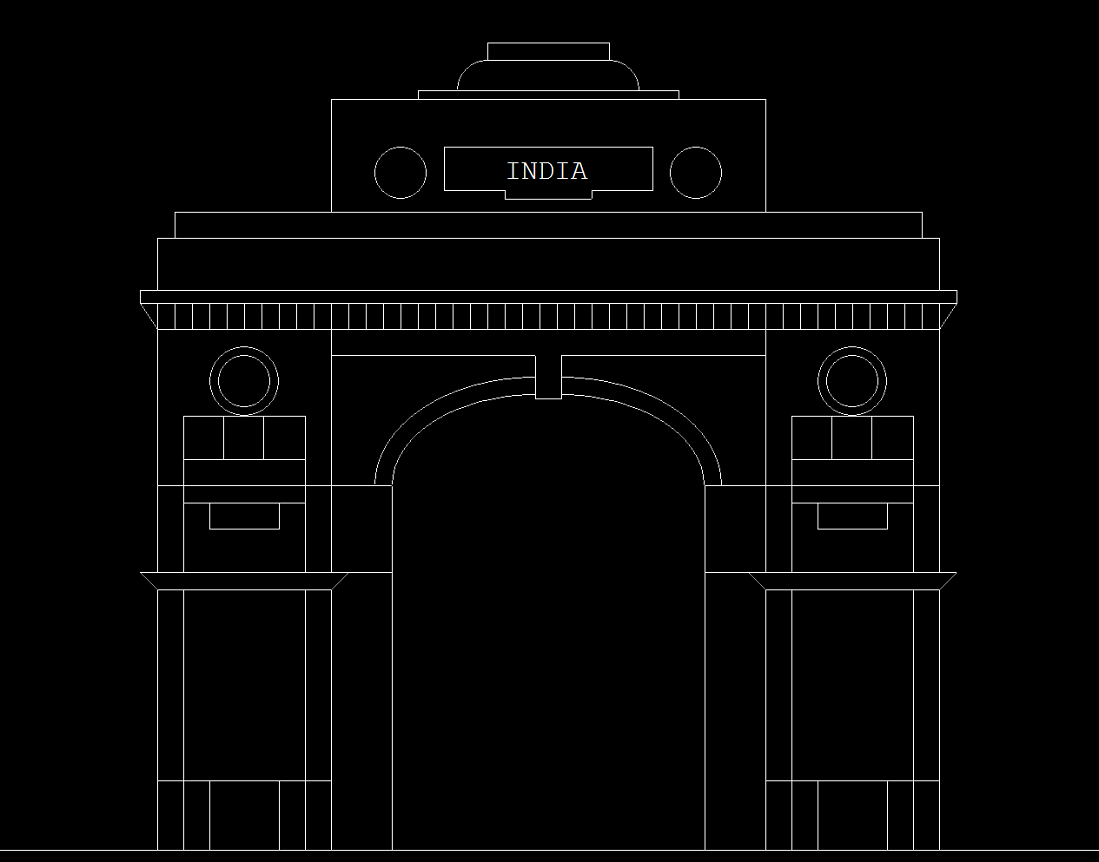Related Articles

# Program to draw India Gate using computer graphics in C

• Last Updated : 07 Jul, 2021

p>In C graphics, the graphics.h functions are used to draw different shapes like circles, rectangles, etc, display text(any message) in a different format (different fonts and colors). By using graphics.h one can make programs, animations, and also games.

Function Used:

Take a step-up from those "Hello World" programs. Learn to implement data structures like Heap, Stacks, Linked List and many more! Check out our Data Structures in C course to start learning today.
• rectangle(l, t, r, b): A function from graphics.h header file which is used to draw a rectangle from left(l) to right(r) and from top(t) to bottom(b).
• line(a1, b1, a2, b2): A function from graphics.h header file which is used to draw a line from (a1, b1) point to (a2, b2) point.
• circle( a, b, r): A function from graphics.h header file which is used to draw a circle with (a, b) as the center and r as a radius.
• outtextxy(int x, int y, char *string): A function from graphics.h header file by which one can print any statements where, x, y are the coordinates of the point and, the third argument contains the address of the string to be displayed.
• settextstyle(int font, int direction, int font_size): A function from graphics.h header file by which one can create the style of the printable text where the font argument specifies the font of the text. The direction can be HORIZ_DIR (Left to right) or VERT_DIR (Bottom to top).
• ellipse(int x, int y, int start_angle, int end_angle, int x_radius, int y_radius): A function from graphics.h header file where x, y are the location of the ellipse. x_radius and y_radius decide the radius of form x and y. start_angle is the starting point of the angle and end_angle is the ending point of the angle. The value of the angle can vary from 0 to 360 degrees.
• arc(x, y, start_ang, end_ang, r): A function from graphics.h header file which draws an arc with (x, y) as the center, start_ang is its starting angle and end_ang is its ending angle, and r as a radius.

Approach: Follow the steps below to solve this problem:

• First, define the baseline using the line() function.
• Call for the left() function to define and implement decorations on the left side of the India Gate. In the left() function, first, define a rectangle using the rectangle() function that will act as the lower base rectangle and that will be decorated using the line() function. Then implement another rectangle, which is the upper side rectangle, using the rectangle() function. Here, decorate it using a line() and circle() function.
• Call for the right() function to perform the same steps as discussed above for the left side with the changed coordinates.
• Call for the mid() function to implement decoration of the middle part. Here, implement some arcs by using the ellipse() function. Also, use the line() function to make some other decorations also.
• Call for the up() function. Here, implement some rectangles using the rectangle() function. Here, use the while loop to implement continuous lines with a line() function for decoration purposes. Here, implement some different sized rectangles for decorating purposes.
• In the up() function, one makes some decoration in a rectangle with a circle() and line() function. In the middle of the special rectangle which is built with a line() function, write ‘INDIA’ which is generally seen in the real one. Also, two arcs are implemented with the arc() function to make the upper decoration.

Below is the implementation of the above approach:

## C

 `// C program for the above approach`` ` `#include ``#include ``#include `` ` `// Used Function Declaration``void` `left();``void` `right();``void` `mid();``void` `up();`` ` `void` `up()``{``    ``int` `u = 520;``    ``rectangle(500, 370, 1400, 400);`` ` `    ``// Defining A loop To Draw``    ``// Many Lines``    ``while` `(u <= 1400) {``        ``line(u, 370, u, 400);``        ``u = u + 20;``    ``}`` ` `    ``rectangle(480, 355, 1420, 370);``    ``line(500, 400, 480, 370);``    ``line(1400, 400, 1420, 370);``    ``rectangle(500, 355, 1400, 295);``    ``rectangle(520, 265, 1380, 295);`` ` `    ``// Main Rectangle To Write``    ``// India``    ``rectangle(700, 135, 1200, 265);``    ``circle(780, 220, 30);``    ``circle(1120, 220, 30);``    ``line(830, 190, 1070, 190);``    ``line(830, 190, 830, 240);``    ``line(830, 240, 900, 240);``    ``line(1070, 190, 1070, 240);``    ``line(1070, 240, 1000, 240);``    ``line(1000, 240, 1000, 250);``    ``line(900, 240, 900, 250);``    ``line(900, 250, 1000, 250);`` ` `    ``// Writing India``    ``settextstyle(8, 0, 4);``    ``outtextxy(900, 200, ``"INDIA"``);``    ``rectangle(800, 125, 1100, 135);``    ``rectangle(880, 70, 1020, 90);``    ``arc(880, 125, 90, 180, 35);``    ``arc(1020, 125, 0, 90, 35);``}`` ` `// Function to draw the middle part``// of the India Gate``void` `mid()``{``    ``line(700, 400, 1200, 400);``    ``line(700, 430, 935, 430);``    ``line(965, 430, 1200, 430);``    ``line(935, 480, 935, 430);``    ``line(965, 480, 965, 430);``    ``line(935, 480, 965, 480);`` ` `    ``// Left side arcs``    ``ellipse(935, 580, 90,``            ``180, 165, 105);``    ``ellipse(935, 580, 90,``            ``180, 185, 125);`` ` `    ``// Right Side Arcs``    ``ellipse(965, 580, 0,``            ``90, 165, 105);``    ``ellipse(965, 580, 0,``            ``90, 185, 125);`` ` `    ``// Left Side Vertical Line``    ``line(770, 1000, 770, 580);`` ` `    ``// Right Side Vertical Line``    ``line(1130, 1000, 1130, 580);``}`` ` `// Function to draw the left part``// of the India Gate``void` `left()``{``    ``// Left Base Rectangle``    ``rectangle(500, 700,``              ``700, 1000);`` ` `    ``// Lower Inner Rectangle``    ``rectangle(530, 700,``              ``670, 1000);``    ``rectangle(560, 920,``              ``640, 1000);``    ``line(500, 920, 700, 920);``    ``line(500, 700, 700, 700);``    ``line(480, 680, 770, 680);``    ``line(500, 700, 480, 680);``    ``line(700, 700, 720, 680);`` ` `    ``// Left Upper Rectangle``    ``rectangle(500, 400,``              ``700, 680);`` ` `    ``// Upper Inner Rectangle``    ``rectangle(530, 500,``              ``670, 680);``    ``line(530, 550, 670, 550);``    ``line(576, 500, 576, 550);``    ``line(622, 500, 622, 550);``    ``line(500, 580, 770, 580);``    ``line(530, 600, 670, 600);``    ``rectangle(560, 600,``              ``640, 630);``    ``circle(600, 460, 40);``    ``circle(600, 460, 30);``}`` ` `// Function to draw the right part``// of the India Gate``void` `right()``{``    ``// Right Base Rectangle``    ``rectangle(1200, 700,``              ``1400, 1000);`` ` `    ``// Lower Inner Rectangle``    ``rectangle(1230, 700,``              ``1370, 1000);``    ``rectangle(1260, 920,``              ``1340, 1000);``    ``line(1200, 920, 1400, 920);``    ``line(1200, 700, 1400, 700);``    ``line(1130, 680, 1420, 680);``    ``line(1200, 700, 1180, 680);``    ``line(1400, 700, 1420, 680);`` ` `    ``// Right Upper Ractangle``    ``rectangle(1200, 400,``              ``1400, 680);`` ` `    ``// Upper Inner Rectangle``    ``rectangle(1230, 500,``              ``1370, 680);``    ``line(1230, 550, 1370, 550);``    ``line(1276, 500, 1276, 550);``    ``line(1322, 500, 1322, 550);``    ``line(1130, 580, 1400, 580);``    ``line(1230, 600, 1370, 600);``    ``rectangle(1260, 600,``              ``1340, 630);``    ``circle(1300, 460, 40);``    ``circle(1300, 460, 30);``}`` ` `// Driver Code``void` `main()``{``    ``int` `gd = DETECT, gm;`` ` `    ``// Initialize of gdriver with``    ``// DETECT macros``    ``initgraph(&gd, &gm,``              ``"C:\\turboc3\\bgi"``);`` ` `    ``// Base Line``    ``line(200, 1000, 2000, 1000);`` ` `    ``// Function Call``    ``left();`` ` `    ``// Function Call``    ``right();`` ` `    ``// Function Call``    ``mid();`` ` `    ``// Function Call``    ``up();`` ` `    ``// Holding The Screen For A While``    ``getch();`` ` `    ``// Close the initialized gdriver``    ``closegraph();``}`

Output:My Personal Notes arrow_drop_up